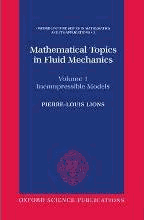# Mathematical Topics in Fluid Mechanics: Volume 1: Incompressible Models by Lions, Pierre-Louis

• R 1,500.00
Unit price per
• Save R 867.90
Tax included.

ISBN: 9780198514879

Oxford University Press | 9780198514879

Hardback | 252 pages

One of the most challenging topics in applied mathematics over the past decades has been the developent of the theory of nonlinear partial differential equations. Many of the problems in mechanics, geometry, probability, etc lead to such equations when formulated in mathematical terms. However, despite a long history of contributions, there exists no central core theory, and the most important advances have come from the study of particular equations and classes of

equations arising in specific applications. This two volume work forms a unique and rigorous treatise on various mathematical aspects of fluid mechanics models. These models consist of systems of nonlinear partial differential equations like the incompressible and compressible Navier-Stokes

equations. The main emphasis in Volume 1 is on the mathematical analysis of incompressible models. After recalling the fundamental description of Newtonian fluids, an original and self-contained study of both the classical Navier-Stokes equations (including the inhomogenous case) and the Euler equations is given. Known results and many new results about the existence and regularity of solutions are presented with complete proofs. The discussion contiatns many interesting insights and

remarks. The text highlights in particular the use of modern analytical tools and methods and also indicates many open problems. Volume 2 will be devoted to essentially new results for compressible models. Written by one of the world's leading researchers in nonlinear partial differential equations,

Mathematical Topics in Fluid Mechanics will be an indispensable reference for every serious researcher in the field. Its topicality and the clear, concise, and deep presentation by the author make it an outstanding contribution to the great theoretical problems in science concerning rigorous mathematical modelling of physical phenomena. Pierre-Louis Lions is Professor of Mathematics at the University of Paris-Dauphine and of Applied Mathematics at the Ecole Polytechnique.